Welcome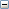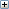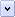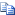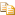GetPolyLinesAndCurves Method (IView)

Gets the arcs, ellipses, splines, and polylines in the view with an option to include or exclude crosshatch lines.

.NET Syntax

Visual Basic (Declaration)
Function GetPolyLinesAndCurves( _
ByVal CrossHatchOption As System.Short _
) As System.Object
Visual Basic (Usage)
Dim instance As IView
Dim CrossHatchOption As System.Short
Dim value As System.Object

value = instance.GetPolyLinesAndCurves(CrossHatchOption)
C#
System.object GetPolyLinesAndCurves(
System.short CrossHatchOption
)
C++/CLI
System.Object^ GetPolyLinesAndCurves(
&   System.short CrossHatchOption
)

Parameters

CrossHatchOption
• 0 = include crosshatch lines
• 1 = exclude crosshatch lines

• 2 = include only crosshatch lines

• Return Value

Array of lines and parameters in the view (see Remarks)

Remarks

The difference between this method and IView::GetPolylines7 is that splines and ellipses returned by this method are returned as equation parameters, which are more precise definitions.

This method returns data for all visible solid model edges in the drawing view. This includes these types of edges:

• 1= arcs: Returned using a center point, radius, and start and stop location.

• 1 = circles: Returned using a center point, radius, and start and stop location.

• 2 = ellipses: Returned as equation parameters.

• 3 = splines: Returned as equation parameters.

• 0 = straight lines: Returned as tessellated polylines, but because the edges are straight, there is no loss of data in the approximation.

This method returns data for all visible solid model edges in the drawing view. This includes these types of edges:

• 1= arcs: Returned using a center point, radius, and start and stop location.

• 1 = circles: Returned using a center point, radius, and start and stop location.

• 2 = ellipses: Returned as tessellated polylines.

• 3 = splines: Returned as tessellated polylines.

• 0 = straight lines: Returned as tessellated polylines, but because the edges are straight, there is no loss of data in the approximation.

For each arc:

• first double gives the type

• next double gives the number of doubles required to hold arc geometry ( center, startPt, endPt, normal ) == 12

• next three doubles give the coordinates of center

• next three doubles give the coordinates of startPt

• next three doubles give the coordinates of endPt

• next three doubles give the coordinates of the normal

• next six doubles are line attributes:

• LineColor = polyline color. This value is determined either by the explicitly set value or by the layer that the polyline is on.

• LineStyle = value combines polyline font and weight information. This value can be used as an input to GetLineFontInfo and GetLineFontName. If this value is -1, then the user has probably modified the line display manually in the drawing and you should use the LineFont and LineWeight return values to recreate the exact polyline style.

• LineFont = value is used for polyline font information. This value can be used as an input to the GetLineFontInfo2 and GetLineFontName2 functions. This value will only be valid if LineStyle is -1.

• LineWeight = polyline weight where Thin = 0.0, Normal = 1.0, Thick = 2.0. This value will only be valid if LineStyle is -1.

• LayerID = integer value indicating which layer holds this polyline. The ILayer object can be obtained by passing this integer value to ILayerMgr::GetLayerById.

• LayerOverride = integer with bit flags set to determine which properties, if any, have been overridden with respect to the Layer default properties. If the bit value is set, then the specific property or properties have been overridden. The bit indicators are: color = 0x1, style = 0x2, and width = 0x4. Therefore, if LayerOverride was returned as 3, you know the color and style have been specifically set for this item and may not match the default values associated with this item's layer.

• next double is the number of points lying on the arc

• next ( numPoints * 3 ) doubles are the coordinates of points lying on the arc

For each ellipse:

• first double gives the type

• next double gives the number of doubles required to hold ellipse geometry ( center, unit vector along majorAxis, unit vector along minor axis, major radius, minor radius, startPt, endPt, normal ) == 17

• next three doubles give the coordinates of center

• next three doubles give the coordinates of unit vector along major axis

• next three doubles give the coordinates of unit vector along minor axis

• next doubles gives the major radius

• next double gives the minor radius

• next three doubles give the coordinates of startPt

• next three doubles give the coordinates of endPt

• next six doubles are line attributes (see description of line attributes in previous bulleted item)

• next double is the number of points lying on the ellipse

• next ( numPoints * 3 ) doubles are the coordinates of points lying on the ellipse

For each spline

• first double gives the type

• next double gives the number of doubles required to hold the information about the spline geometry ( 5 + numCtrlPts* numDims + numKnot ); if the spline is rational, numDims ==4 otherwise numDims ==3

• next double gives degree = order -1

• next double gives whether the spline is rational (1) or non-rational (0)

• next double gives numDims ( i.e., number of coordinates associated with each control point; if the spline is rational , these are four, else three)

• next double gives the number of control points of the spline

• next double gives the number of knots on the spline

• next ( numCtlPoints*numDims ) number of doubles are the coordinates of control points; each control point has numDims number of coordinates

• next "numKnots" number of doubles; the knots on the spline

• next six doubles are line attributes (see description of line attributes in previous bulleted item)

• next double is the number of points lying on the spline

• next ( numPoints * 3 ) doubles are the coordinates of points lying on the spline

• For each polyline

• first double gives the type

• next double holds value 0.0 as there is no geometry information for a polyline

• next six doubles are line attributes (see description of line attributes in previous bulleted item)

• next double is the number of points lying on the polyline

• next ( numPoints * 3 ) doubles are the coordinates of points lying on the polyline

This method also lets you include or exclude crosshatch lines. This method does not return data for sketch segments added by a user in the drawing view.

 To get... Call... Size of array needed to hold this data Items that were sketched in this drawing view

 If... Then... Display mode of the view is: Shaded Shaded with Edges Draft Quality Fast HLR/HLV A value is not returned. Use IView::SetDisplayMode3 to change Shaded or Shaded with Edges mode to Wireframe, Hidden Lines Removed (HLR), or Hidden Lines Visible (HLV), and then get the polylines. Changes are made to the parts or assemblies shown in this drawing Polylines are only generated that are in the visible viewing bounds when the drawing is opened. Drawing is already open All polylines in the drawing are generated. If you open a drawing that is zoomed in to a particular region, then the polylines that are outside the zoomed region do not exist if the parts or assemblies shown in this drawing have been changed. To force the generation of all possible polyline data, call IModelDoc2::ViewZoomtofit2.

Availability

SOLIDWORKS 2006 FCS, Revision Number 14.0

Provide feedback on this topic

SOLIDWORKS welcomes your feedback concerning the presentation, accuracy, and thoroughness of the documentation. Use the form below to send your comments and suggestions about this topic directly to our documentation team. The documentation team cannot answer technical support questions. Click here for information about technical support.

* Required

 *Email: Subject: Feedback on Help Topics Page: GetPolyLinesAndCurves Method (IView) *Comment: * I acknowledge I have read and I hereby accept the privacy policy under which my Personal Data will be used by Dassault Systèmes

Print Topic

Select the scope of content to print:

x

We have detected you are using a browser version older than Internet Explorer 7. For optimized display, we suggest upgrading your browser to Internet Explorer 7 or newer.

Never show this message again
x

Web Help Content Version: API Help (English only) 2016 SP05

To disable Web help from within SOLIDWORKS and use local help instead, click Help > Use SOLIDWORKS Web Help.

To report problems encountered with the Web help interface and search, contact your local support representative. To provide feedback on individual help topics, use the “Feedback on this topic” link on the individual topic page.